Python Tutorials

# Write your first python Program | Hello World

In the previous tutorial, we had created a project with the name  “HelloWorld”. Now we learn how to write the first Python Program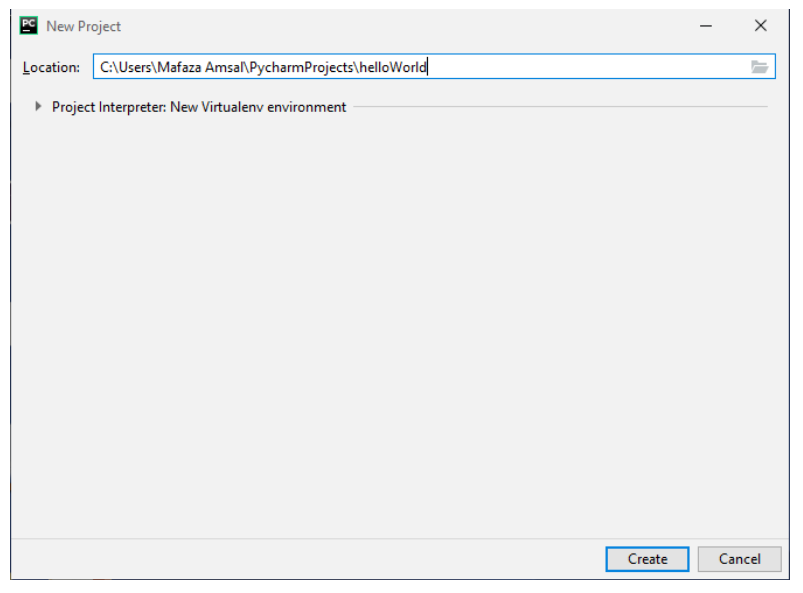### Step 1:

Click on the file on the top left of the PyCharm editor and select new as shown in the editor below.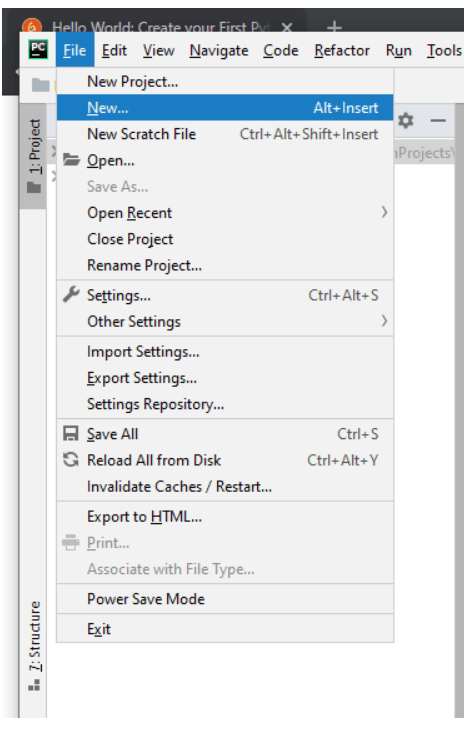### Step 2:

Then select “Python file” as shown below.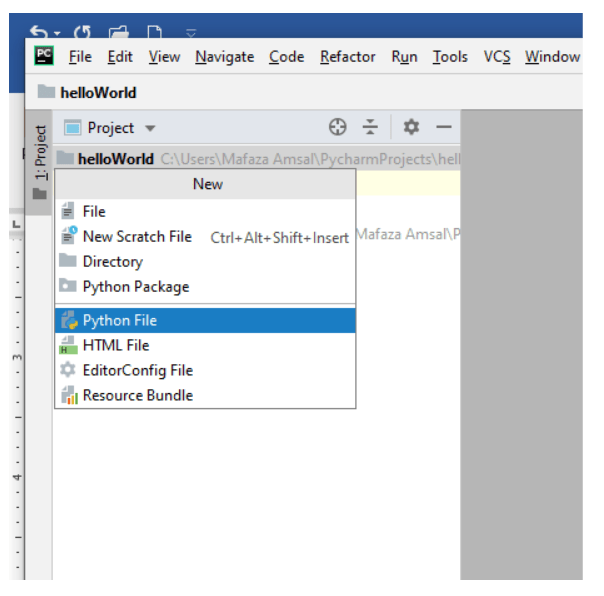### Step 3:

write the name of the file “HelloWorld” and press enter.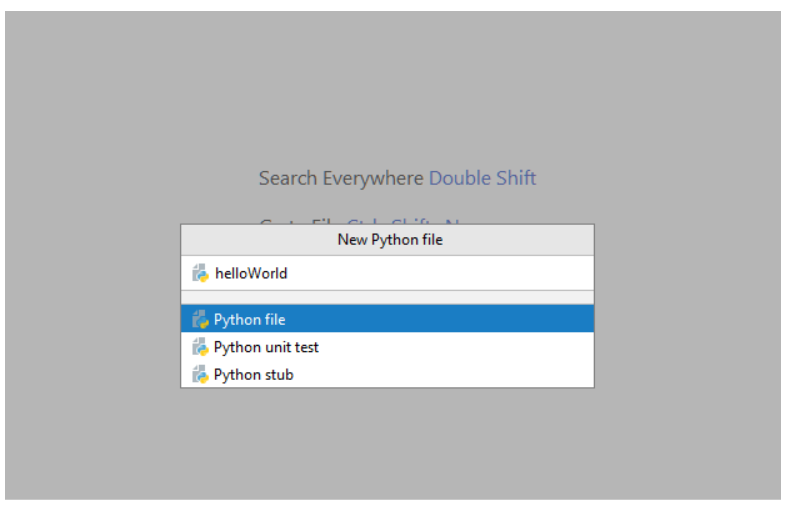### Step 4:

Now you have the space to write your first program. Write “print(“hello world”)”### Step 5:

Right-click on the white space and menu will be shown.

Then select “Run ‘HelloWorld’”### Step 6:

Below a terminal will open and the output of the program will be shown.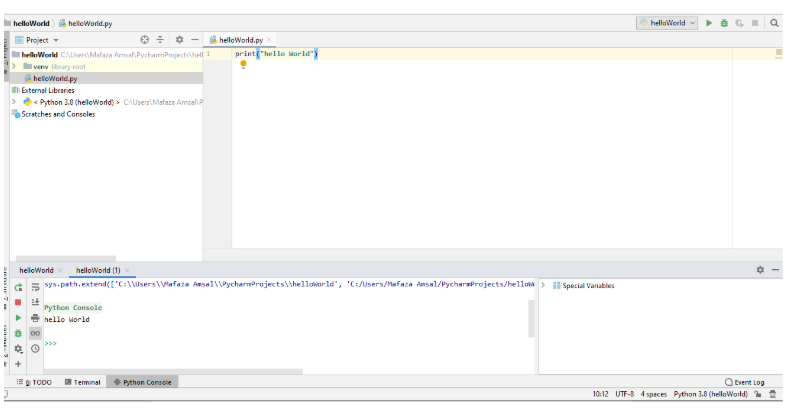Congratulations you have successfully written your first program.

## Rectangle Example

Now let us see how we can simply draw a rectangle using print() statement. Python executes the code line by line. We are going to use ‘*’ to print the rectangle. Simply write the program as shown below.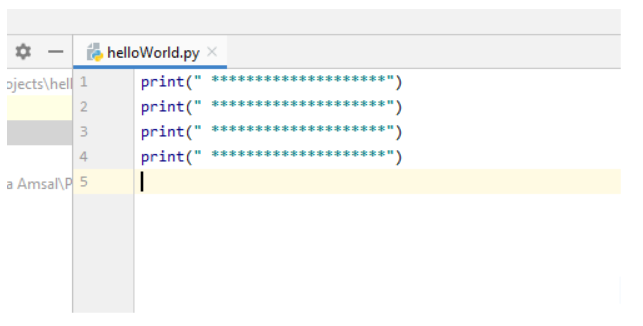Then repeat step 5 to run the program.

You have successfully drawn the rectangle.In the upcoming tutorials, we will see how we can reduce the repetitions of writing the same lines of code.

## How to print blank lines

Python allows three ways to print blank lines.

1. print()
2. print(“ ”)
3. print(“write backward slash n at end of text\n”)

Let us try all three ways to print blank lines.
Line 1 and 2 have no blank space between them.
Line 3 and 5 prints a blank space.
Line 6 uses “\n” at the end to print the blank space.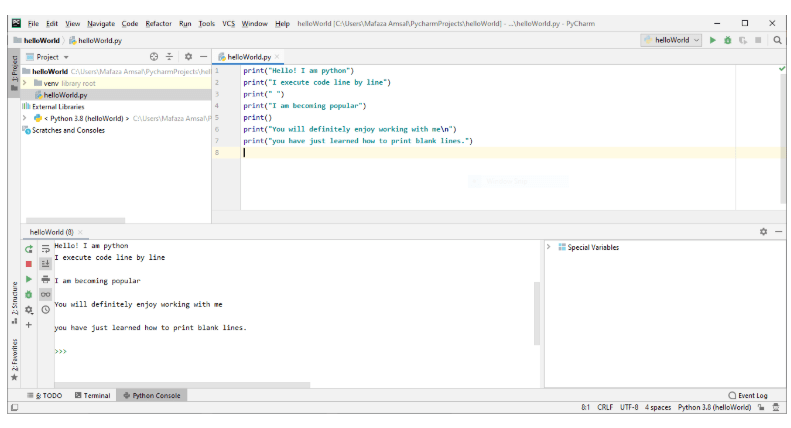## Use of end in print()

As the print is a function, we can pass an “end” optional parameter. If you don’t understand parameters don’t worry, you will understand in the upcoming function tutorials.  “end” is used to tell the print statement how we want to end the print line. In the previous examples, we used the default end parameter which automatically end the line.

Let’s understand with an example.

Line 1 and 2 are simple print statements as we use print statement previously.
Line 3 prints a blank line.
Line 4 uses end=” “means “end this print line with a blank whitespace not with blank line”.
Line 7 uses end=”—” means “end this print line with —”.

See the difference in the output.